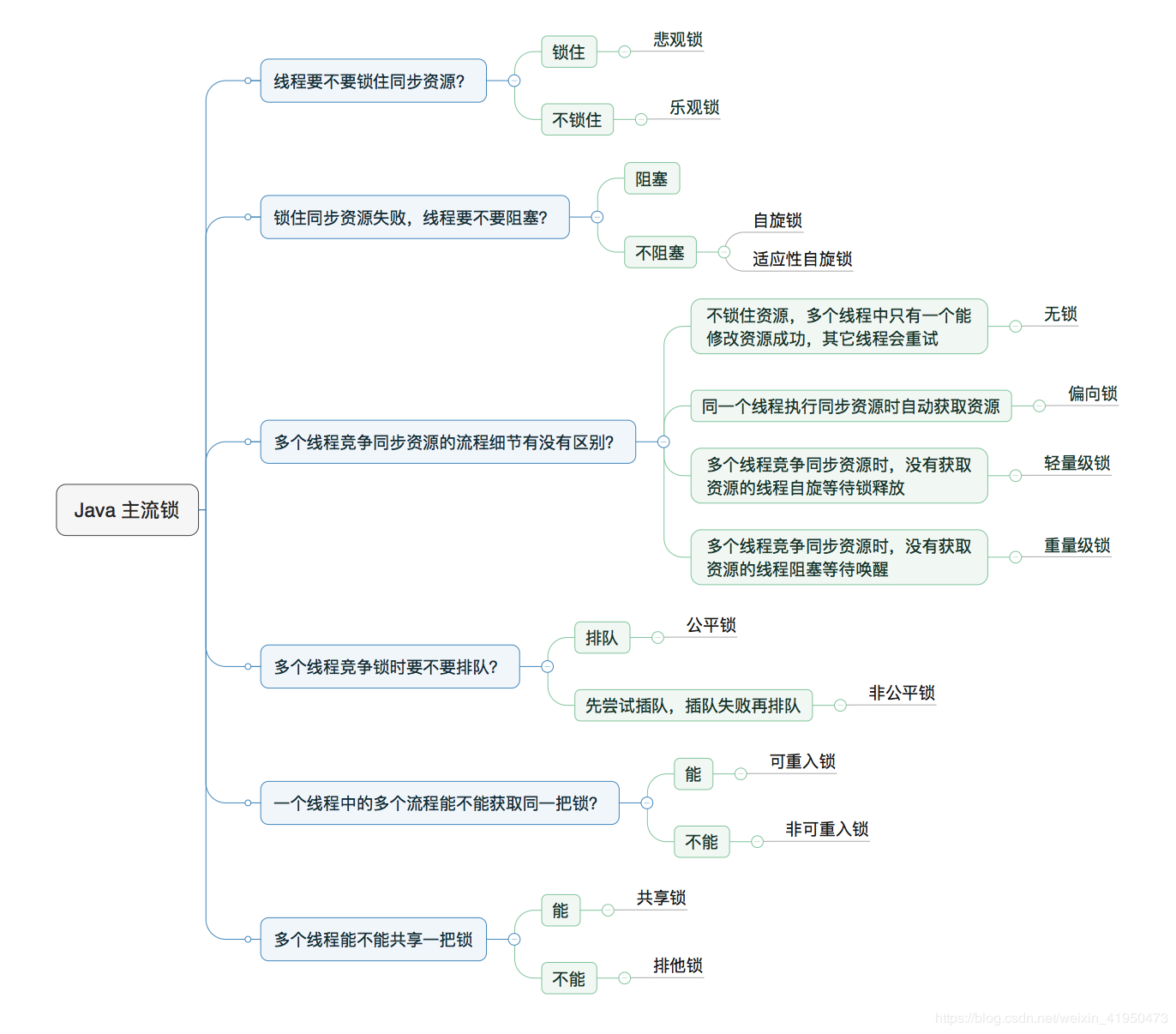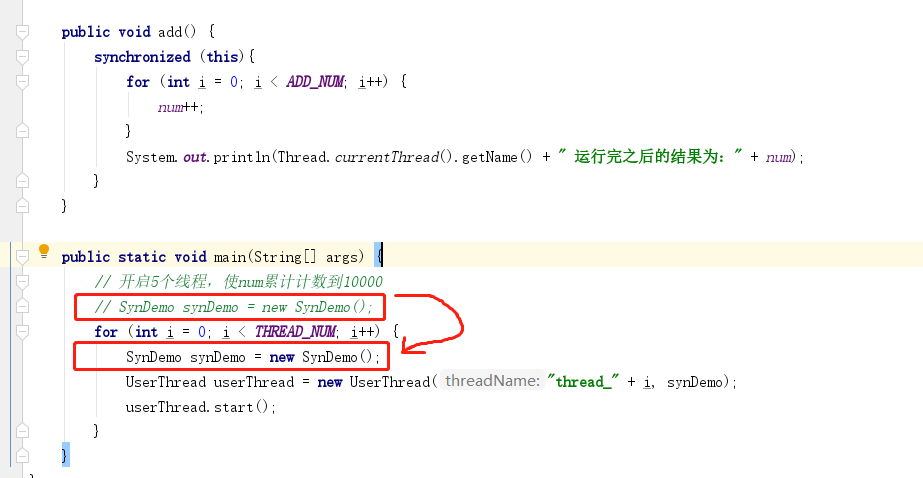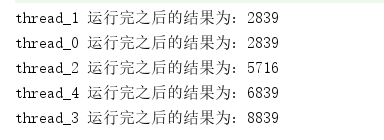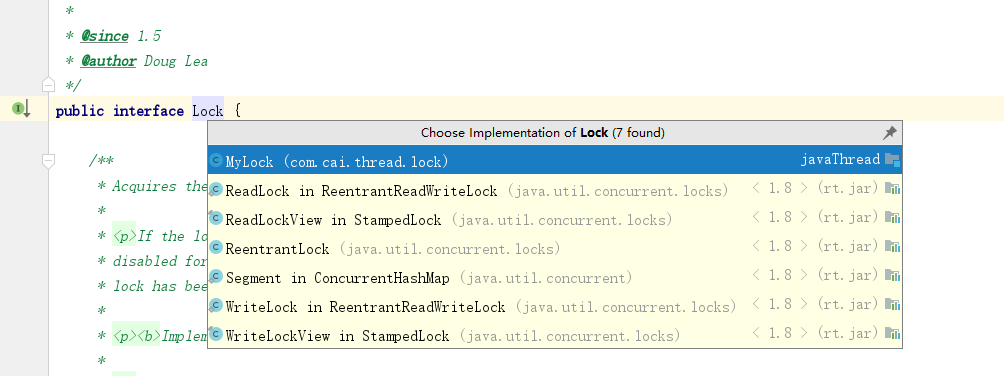# Java线程知识：二、锁的简单使用

### 锁的初步认识### synchronized 内置锁(隐式锁)

#### 1、从语法维度上来讲，`synchronized`一共有三种用法：

• 静态方法上加关键字
`````` public static synchronized void add(){}
``````
• 实例方法上加关键字
``````public synchronized void add(){}
``````
• 方法中使用同步代码块
``````public void add(){
synchronized(this){}
}
``````

``````/**
* @author cai
*/
public class SynDemo {
private static int num = 0;

private static final int ADD_NUM = 2000;
private static final int THREAD_NUM = 5;

private SynDemo synDemo;

this.synDemo = synDemo;
}

@Override
public void run() {
}
}

for (int i = 0; i < ADD_NUM; i++) {
num++;
}
+ " 运行完之后的结果为：" + num);
}

public static void main(String[] args) {
// 开启5个线程，使num累计计数到10000
SynDemo synDemo = new SynDemo();
for (int i = 0; i < THREAD_NUM; i++) {
}
}
}
``````1.1 实例方法上加关键字

``````/**
* 在实例方法 （普通方法） 上加关键字
*/
for (int i = 0; i < ADD_NUM; i++) {
num++;
}
}
``````1.2 静态方法上加关键字

``````/**
* 在静态方法上加关键字
*/
public static synchronized void add() {
for (int i = 0; i < ADD_NUM; i++) {
num++;
}
}
``````1.3 方法中使用同步代码块

``````public void add() {
synchronized (this){
for (int i = 0; i < ADD_NUM; i++) {
num++;
}
}
}
``````#### 2、从synchronized锁的是谁的维度来讲，一共有两种情况：

2.1 对象锁``````/**
* @author cai
*/
public class SynDemo {
private static int num = 0;

private static final int ADD_NUM = 2000;
private static final int THREAD_NUM = 5;

// 共享的对象
private static SynDemo synDemo = new SynDemo();

this.synDemo = synDemo;
}*/

}
@Override
public void run() {
}
}

synchronized (synDemo){
for (int i = 0; i < ADD_NUM; i++) {
num++;
}
+ " 运行完之后的结果为：" + num);
}

}

public static void main(String[] args) {
// 开启5个线程，使num累计计数到10000
// SynDemo synDemo = new SynDemo();
for (int i = 0; i < THREAD_NUM; i++) {
//            SynDemo synDemo = new SynDemo();
}
}
}
``````2.2 类锁

``````public void add() {
//        synchronized (this) {
synchronized (SynDemo.class){
for (int i = 0; i < ADD_NUM; i++) {
num++;
}
+ " 运行完之后的结果为：" + num);
}

}

public static void main(String[] args) {
// 开启5个线程，使num累计计数到10000
// SynDemo synDemo = new SynDemo();
for (int i = 0; i < THREAD_NUM; i++) {
SynDemo synDemo = new SynDemo();
}
}
```````````` public synchronized void add() {
//        synchronized (this) {
//        synchronized (SynDemo.class){
for (int i = 0; i < ADD_NUM; i++) {
num++;
}
+ " 运行完之后的结果为：" + num);
//        }

}

public static void main(String[] args) {
// 开启5个线程，使num累计计数到10000
// SynDemo synDemo = new SynDemo();
for (int i = 0; i < THREAD_NUM; i++) {
SynDemo synDemo = new SynDemo();
}
}
````````````public static synchronized void add() {
//        synchronized (this) {
//        synchronized (SynDemo.class){
for (int i = 0; i < ADD_NUM; i++) {
num++;
}
+ " 运行完之后的结果为：" + num);
//        }

}
``````• `public synchronized void add(){}``synchronized(this){}``private SynDemo synDemo = new SynDemo(); synchronized(synDemo){}`这三种写法中的`synchronized`锁住的都是对象，即对象锁
• `public static synchronized void add(){}``synchronized(SynDemo.class){}`这两种写法中的`synchonized`锁住的都是类，即类锁
• 建议： 在日常工作或学习中，使用代码块加锁的方式。

### Lock 显示锁

`synchronized`不同，`Lock``JDK1.5`为我们提供的一个`api`，所以它与`synchronized`一明一暗，被称为显示锁

`Lock`作为一个接口，有着多个实现类：`ReadLock``ReentrantLock``WriteLock` ……``````private static Lock lock = new ReentrantLock();

// 拿到锁
lock.lock();
try {
for (int i = 0; i < ADD_NUM; i++) {
num++;
}
+ " 运行完之后的结果为：" + num);
} finally {
// 释放锁
lock.unlock();

}
}
``````

`synchronized`不同，`lock`并没有类锁和对象锁的分类，它的用法也是非常的简单，`lock()`方法是当前线程拿到锁，`unlock()`方法是当前线程释放锁。是的，`lock``synchronized`最大的不同就是`lock`需要线程自己去释放锁，而`synchronized``JVM`帮我们释放锁。如果当前拿到锁的线程不及时的调用`unlock()`方法时，程序将不会终止，所有的线程都会卡在方法外。``````private static Lock lock = new ReentrantLock();

// 拿到锁
lock.lock();
try {
for (int i = 0; i < ADD_NUM; i++) {
num++;
}
+ " 运行完之后的结果为：" + num);
} finally {
// 释放锁
//            lock.unlock();

}
}
``````• 使用`lock`锁时，必须在`try{}`代码块之前调用`lock()`方法，并在`finally{}`代码块中调用`unlock()`方法及时的释放锁。

### 最后

`synchronized``Lock`不论在本质还是用法上面都有很多的不同，不是一两句就能讲清楚的，在以后的随笔中，我会逐步的去分享`Lock`中的各种方法，会将`sychronized``Lock`的不同做个最后的总结，这也是非常重要的一个知识点。

Java线程知识：二、锁的简单使用

[C#] （原创）一步一步教你自定义控件——01，TrackBar